Discover and enable the integrations you need to solve identityAuth0 Marketplace# Birthday Attacks, Collisions, And Password Strength

Is there a point where a password is so strong it stops making sense?

March 23, 2021

## The Problem With Hashing Functions

We already talked about unnecessarily long passwords from a practical point of view, but what does the math say about it? To discuss that, we need to understand the key properties of hashing functions. Hashing functions are:

• Deterministic (it always produces the same output given the same input), and
• Irreversible (it’s impossible to convert hash values back to plaintext)

However, that poses a problem. How can we guarantee that each output will be unique so that two different passwords don’t map to the same output?

Well, we can’t, because hash functions will output a fixed-length hash. The number of possible hash values will depend on the function used:

• MD5 can output 2^128 different hash values,
• SHA-1 can output 2^160 different hash values,
• bcrypt can output 2^192 different hash values and so forth.

Since inputs are infinite, some of them will invariably be mapped to the same hash output.

NOTE:

While some applications limit their user’s password length, in theory, the input can be infinite.

In mathematics, this is known as the Pigeonhole Principle, and in cryptography, hash collisions are one example of its manifestation.

### Hash Collisions

Why does this pose a threat to you, the user? At the end of the day, the goal of the attacker is not to find your password but a password that maps to the hash that’s stored on the server.

If another string maps to the same hash output as your password, it would be authenticated by the server.

So it begs the question: how likely is it for two passwords to have the same hash in the first place? The answer to this question is better illustrated by a famous problem in probability theory known as the Birthday Problem.

## The Birthday Problem

The Pigeonhole principle states that if n items are put into m containers, with n > m, then at least one container must contain more than one item.

For example, we have around 7.5 billion people on the planet (“n items”), but we can only be born in 365 days of the year (“m containers”).

There is a famous application of the Pigeonhole Principle in mathematics related to this problem known as the Birthday Problem. It can be stated as such:

How many people must be in a room such that the chance of two people sharing a birthday is 50%? We’ll exclude leap years for simplicity. We’ll also assume that the likelihood of a person being born in each of the 365 days is equal. (This is not true; people are slightly more likely to be born in August and September.)

If you weren’t familiar with this problem before, you might be surprised to learn that the answer is only 23 people.

### Math Behind The Problem

Suppose that the chance of two people having a birthday on the same day is P(A), and the chance of two people not having a birthday on the same day is P(B).

Since both probabilities are mutually exclusive (you either have a birthday on the same day as someone else or you don’t), it comes that the chance of two people having a birthday on the same day is P(A) = 1 - P(B).

Since it’s often easier to solve for P(B) in cases like this, we’ll start by solving for P(B). First, we have to find out what’s the chance of not having a matching birthday among the 23 people in the room.

The first person can be born on any of the 365 days, and there would still be no matching birthdays. The second person can only be born on any of the remaining 364 days out of 365, excluding the day the first person was born. The third person can only be born on the remaining 363 days, excluding the other two days the other two people were born, and so on, until the 23rd person: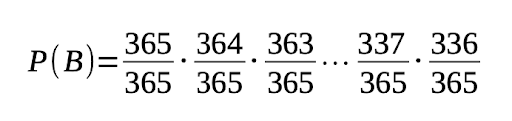That results in ≈ 0.492. Therefore, P(A) = 0.508 or 50.8%. This process can be generalized to a group of N people, where P(N) is the probability of at least two people sharing a birthday: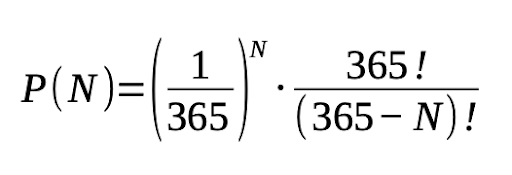Here are the probabilities for different values of N: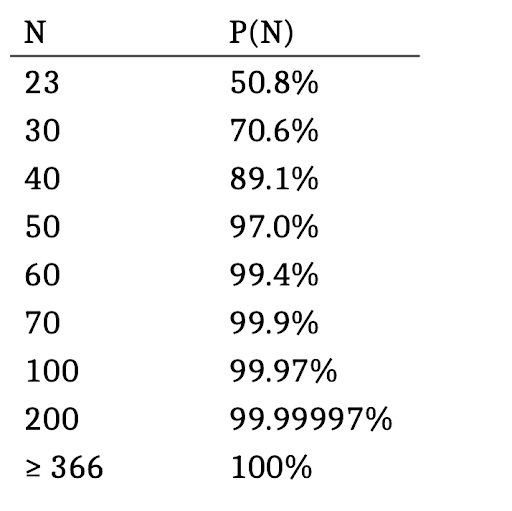Note that because of the Pigeonhole Principle, for any N equal or greater than 366, the probability of a shared birthday is 100%.

This problem is sometimes called the Birthday Paradox because it may seem counter-intuitive that it only takes 23 people for the chance to be around 50% (most people would guess that the chance would be around 183, about half of 365).

How does this relate to hashing functions?

The same thing happens with hashing functions since we are mapping infinite inputs to fixed-size outputs. Let’s use MD5 as an example. The infinite input possibilities will get pigeonholed into 2^128 different possible outputs. What are the chances that there will be hash collisions for a sample space of that size?

### Birthday Problem Applied To Hashing Functions

We can generalize the answer from the Birthday Problem to a sample space of any size. Let’s call H the sample space of a given hash function: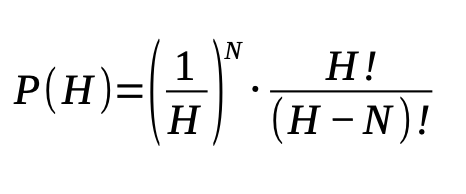If you solve this equation for the sample spaces of different hashing functions, you will see that a collision will always happen after roughly N/2 operations (where N is the size of the sample space in bits).

That means that you stand a 50% chance of finding an MD5 collision (sample space of 2^128 possibilities) after around 2^64 operations and a 50% chance of finding an SHA-1 collision (sample space of 2^160 possibilities) after around 2^80 operations.

As you can see, this is way fewer operations than a brute-force attack. In cryptography, this is called a Birthday Attack.

## What If 1234 Is Mapped To The Same Hash As My Strong Password?

The Birthday Problem is a good party trick because “23 people” is way fewer than you’d expect... But that’s not relevant, is it?

What you want to know is the chance of someone sharing a birthday (hash value) with you. That is, you’re not worried if there are hash collisions in general, but if there are other hashes colliding with the hash value of your password.

### Birthday Problem Revisited

Let’s revisit the Birthday Problem, but this time, instead of looking at the probability of any people among the 23 sharing birthdays, none of which concern you, let’s look at the chance of one of them sharing a birthday with you.

Again, we are going to look at the chance of someone not sharing a birthday with you, P(B’), then take the complement to find out the chance of someone sharing a birthday with you, P(A’).

Assume you are born on any given day out of 365. The second person can be born on any of the other 364 days, excluding your birthday. The third person can also be born on any of the other 364 days. And so forth, until the N-th person.

So the chance of N people not sharing a birthday with you is: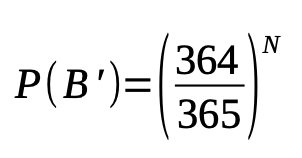For N = 23 people, we have that: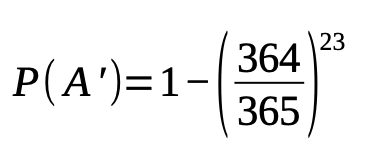Which is about 6.1%. For a >50% chance of one person in a room of N people being born on the same day as you, you’d need 253 people in the room.

This number is much greater than 183 (about half of 365) because it’s likely that some of those people will share birthdays among themselves on different days than yours, so you’d need a few more people to account for that.

In fact, 253 is the number of all pair arrangements that you can make among 23 people, so the amount of people necessary is the same. But this time, we are looking for one specific pair among 253 instead of any pair.

This problem can be generalized for different hashing functions by the following equation: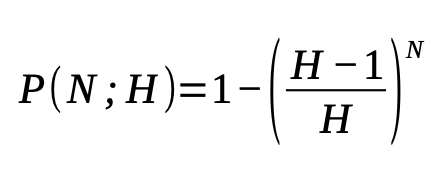So what is the chance that an attacker would be able to get lucky and find a weak password that has the same hash as your strong password?

You’d just have to make P = 0.5 and solve for N.

As an example, if the hashing function is MD5, the attacker would have to go through 2^(127.5) hashes to have a >50% chance of finding a hash that collides with yours, which is more than the theoretical 2^127 operations to brute-force the entire sample space.

This means that the likelihood of 1234 colliding specifically with yours is less likely to happen than the attacker just guessing your password out of all 2^128 possibilities.

Also, just because someone found a collision doesn’t mean they will be able to log into your account: it could be that the matching password is 300-characters long, and they wouldn’t be able to send it in the password field because the application limits passwords to, say, 100 characters.

## Why Password Strength Doesn’t Matter Past a Certain Point

Hashing functions are expected to resist against two things: collisions and preimage attacks.

This is because hashing functions like the MD and SHA families are general-purpose hashing functions. They are also widely used for other purposes, such as verifying file integrity, so attackers being able to forge two different files with the same hash is problematic.

However, as we’ve seen, collisions aren’t much of an issue if we’re talking about securing passwords. This is why Bruce Schneier said SHA-1 is still good to use with HMAC despite it being broken for collisions (in 2020, an attack was able to find SHA-1 collisions in 2^(63.4) operations, much faster than the theoretical 2^80 operations).

NOTE:

You shouldn’t use SHA-1 to store passwords regardless of that because computers can calculate SHA-1 hashes very fast. There are much better functions that make such attacks less effective.

The most desirable propriety in this circumstance is preimage resistance: given a hash value, how hard is it to revert it to the original message?

Assuming there is no known vulnerability in the function itself, there are only two ways to get around preimage resistance: guessing the password or trying all possible hash values until you find a valid hash. The password strength will be no stronger than the weaker of the two.

We already went over on how, most of the time, it’s a better deal for the attacker to try to guess the password because the sample space is almost always smaller since humans aren’t very good at picking passwords.

However, if you choose a set of passwords with a larger sample space than the output of the hash function, it becomes a better deal to just brute-force the entire hash sample space.

## Summarizing

It doesn’t make sense to have a password with a sample space bigger than the space of the hash function used to store your password because of how they work. The strength of your password is capped at the sample size of the hash output.

Naturally, you have no way of knowing how your passwords are stored most of the time, but for all practical purposes, you don’t need passwords longer than 128 bits, even if your passwords are hashed using a function that outputs longer hashes.

There’s also no need to worry about random collisions compromising your password strength. While there is a chance that your strong password shares a hash with a very weak password, that chance is much smaller than the chance of the attacker brute-forcing the entire sample space of the hashing function.